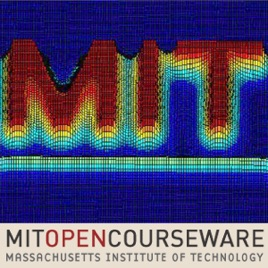29 episodes

This graduate-level course is a continuation of Mathematical Methods for Engineers I (18.085). Topics include numerical methods; initial-value problems; network flows; and optimization.# Mathematical Methods for Engineers II MIT

• Podcasts

This graduate-level course is a continuation of Mathematical Methods for Engineers I (18.085). Topics include numerical methods; initial-value problems; network flows; and optimization.

Lecture 01: Difference Methods for Ordinary Differential Equations

## Lecture 01: Difference Methods for Ordinary Differential Equations

• 44 min
Lecture 02: Finite Differences, Accuracy, Stability, Convergence

## Lecture 02: Finite Differences, Accuracy, Stability, Convergence

• 56 min
Lecture 03: The One-way Wave Equation and CFL / von Neumann Stability

## Lecture 03: The One-way Wave Equation and CFL / von Neumann Stability

• 55 min
Lecture 04: Comparison of Methods for the Wave Equation

## Lecture 04: Comparison of Methods for the Wave Equation

• 52 min
Lecture 05: Second-order Wave Equation (including leapfrog)

## Lecture 05: Second-order Wave Equation (including leapfrog)

• 55 min
Lecture 06: Wave Profiles, Heat Equation / point source

• 52 min